# Diagram

(diff) ← Older revision | Latest revision (diff) | Newer revision → (diff)

in a categoryA mappingof an oriented graphwith set of verticesand set of edgesinto the categoryfor whichand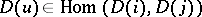if the edgehas source (origin)and target (end). The concept of a diagram inmay also be defined as the image of the mapping, in order to obtain a better visualization of diagrams.

Let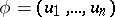be an oriented chain of the graphwith sourceand target, i.e. a non-empty finite sequence of edges in which the source of each edge coincides with the target of the preceding one; also, letdenote the composition of morphismsThe diagramis said to be commutative if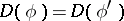for any two oriented chainsandwith identical source and target.

The most frequently encountered forms of diagrams are sequences, triangular diagrams and square diagrams. To define a sequence, the defining graph is taken to have the form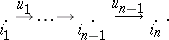The corresponding diagram is represented as follows: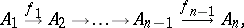where the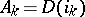are objects in the category, while the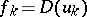are morphisms in this category.

A triangular diagram in a categorycorresponds to the graph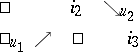and is represented as follows:Commutativity of this diagram means that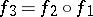.

A square diagram corresponds to the graph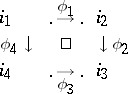and is represented as follows:Commutativity of this diagram means that.

The class of diagrams with a given graphforms a category. A morphism of a diagraminto a diagramis taken to be a family of morphisms, whereruns through the set of vertices of, so that for any edgewith sourceand targetthe condition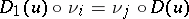is met. In particular, one may speak of isomorphic diagrams. The graphis sometimes referred to as the scheme of a diagram in.

How to Cite This Entry:
Diagram. Encyclopedia of Mathematics. URL: http://encyclopediaofmath.org/index.php?title=Diagram&oldid=46644
This article was adapted from an original article by I.V. Dolgachev (originator), which appeared in Encyclopedia of Mathematics - ISBN 1402006098. See original article